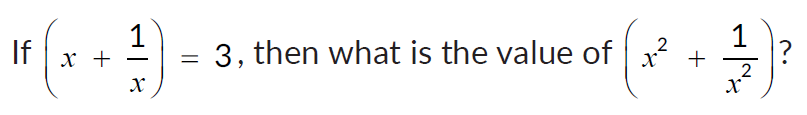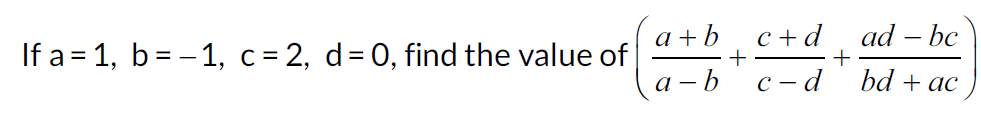Math Concepts
Introduction to Algebra
19k views

 1 Introduction 2 What are algebraic Expressions? 3 Algebraic Identities 4 Properties of Algebra 5 Example Problems 6 Summary 7 About Cuemath 8 Frequently Asked Questions (FAQs) 9 External References

Jan 28, 2021

## Introduction

### What is Algebra?

Algebra is the branch of Maths that uses alphabetical letters to find unknown numbers. These letters are also called variables. The values which are known in the given expression such as numbers are called constants.

Algebra involves the simple operation of mathematics like addition, subtraction, multiplication, and division involving both constant as well as variables. For example, x+10 = 0.

### Introduction to Algebra-PDF

Algebra involves the simple operation of mathematics like addition, subtraction, multiplication, and division involving both constant as well as variables. Here is a downloadable PDF to explore more.

## What are algebraic Expressions?

Algebraic expressions in maths are made up of variables and constants along with operations like addition, subtraction, multiplication, and division.

These expressions can also be named as Terms, which again also called Algebraic Equations. The values which are known in the given expression are represented with the help of variables, constants, and coefficients.

Algebra Expressions covers the simple operation of mathematics like; for example x+10.

Terms related to basic algebra skills are mentioned below.

• Exponent

• Expression

• Polynomial (Monomial, binomial and trinomial)

• Like terms and Unlike terms

• Constants

1. An equation is a statement which implies two same identities separated by the “=” sign.

2. An expression is a group of different terms separated by ‘+’ or ‘-‘ sign.

3. Like terms are those terms whose variables and their exponents are the same.

## Algebraic Identities

Following are the algebraic identities:

• a2 – b2 = (a – b)(a + b)
• (a+b)2= a2 + 2ab + b2
• a2 + b2 = (a – b)2 + 2ab
• (a – b)2 = a2 – 2ab + b2
• (a + b + c)2 = a2 + b2 + c2 + 2ab + 2ac + 2bc
• (a – b – c)2 = a2 + b2 + c2 – 2ab – 2ac + 2bc
• (a + b)3 = a3 + 3a2b + 3ab2 + b3
• (a – b)3 = a3 – 3a2b + 3ab2 – b

## Properties of Algebra

Algebra follows the same set of rules as Number Systems. The order of operations is preserved, and the laws governing addition and multiplication still apply.

However, with the inclusion of variables comes a new set of challenges. For a definitive value for an expression, we need to evaluate an algebraic expression for specific values of the variables, observe how the value of the expression changes as the values of the variables changes.

Like operations with numbers, operations with variables obey certain laws.

The commutative law tells us that we can change the order of the terms while performing addition or multiplication.

• a+b=b+a
• a*b=b*a

The associative law tells us that in addition or multiplication, we can associate the terms or factors as we are comfortable with.

• (a+b)+c=a+(b+c)

Both the commutative law and the associative law apply to either addition or multiplication, but not with both operations together.

The distributive law deals with the combination of addition and multiplication. When a sum is multiplied by value, the value is distributed to each part of the sum.

• a(b+c)=a(b)+a(c)

While solving questions in algebra follow the below steps:

• Identify the relationship between expressions.

• If an expression has a known value, write the other expression in terms of the expression with a known value.

• Substitute the known value for the expression.

• Evaluate the expression.

For Example:

$$2(3x - 7) + 4 (3 x + 2) = 6 (5 x + 9 ) + 3$$
$$6x-14+12x+8=30x+54+3$$
$$18x - 6=30x + 57$$
$$-6=12x +57$$
$$-63=12x$$
$$x= - 63/12$$
$$x= - 21/4$$

## Example Problems

1. Add $$6x + 4y – z + 3$$, $$2y – 3z + 4$$, $$y – 7x + 2z – 1$$ and $$2z – 5x – 6$$.
(A) $$4x – 6y + 2$$     (B) $$–3x + 14y – 3z + 2$$    (C) $$–6x + 7y$$     (D) $$–6x + 6y + z – 4$$

 (C) $$–6x + 7y$$

2.(A) 5     (B) 7     (C) 9     (D) 11

 (B) 7

3. Simplify the expression: $$a – 2b – [5a – 6b – {3a – c + (5a – 2b – 3a – c)}]$$
(A) $$3a – 2a + 2c$$     (B) $$a + 2b – 2c$$     (C) $$4a + 6b$$     (D) $$–2b +2c$$

 (B) $$a + 2b – 2c$$

4. $$3l (l – 4m + 5n)$$ and $$4l (10n – 3m + 2l)$$ are algebraic expressions. What is the algebraic expression obtained when subtracting the first expression from the second expression?
(A) 25lm + 5l2     (B) 25ln – 5l2     (C) 25ln + 5l2     (D) 25lm – 5l2

 (C) 25ln + 5l2

5. Factorize (2a + 3b)2 – (3a – 2b)2.
(A) (5a + b) (5a – b)                      (B) (a + 5b) (a – 5b)
(C) (5a + b) (5b – a)                      (D) (5a + b) (5b + a)

 (C) (5a + b) (5b – a)

6.(A) 1        (B) 0        (C) 3       (D) 2

 (D) 2

7. The perimeter of a triangle is 9x2 – 4x + 3 and the length of two of its sides are 5x2 – 2x + 4 and 2x2 + 2x – 3. Find the length of the third side of the triangle.
(A) 2x2 + 4x – 2                  (B) 2x2 – 4x + 2
(C) 2x2 + 4x – 2                  (D) 2x2 – 4x – 2

 (B) 2x2 – 4x + 2

## Summary

So here we come to the end of this article, let us recapitulate what we learned, So we learned about:

• How to Simplify Algebraic Equations using the BODMAS rule.

• Performing various operations on algebra.

• Solving word problems by reading the statements carefully and writing them down step by step.

Hope you all got relevant information on the topic. You all are free to write comments and like this article.

Cuemath, a student-friendly mathematics and coding platform, conducts regular Online Classes for academics and skill-development, and their Mental Math App, on both iOS and Android, is a one-stop solution for kids to develop multiple skills. Understand the Cuemath Fee structure and sign up for a free trial.

## Who invented algebra?

Abu Ja'far Mohammad Ibn Mousa Al Khwarizmi invented algebra and is also known as the father of algebra.

## What is linear algebra?

A branch of mathematics that is concerned with mathematical structures closed under the operations of addition and multiplication includes the theory of systems of linear equations, matrices, determinants, vector spaces, and linear transformations.

## What is a function in algebra?

A function is a relation for which each value from the set of the first components of the ordered pairs is associated with exactly one value from the set of second components of the ordered pair.

Related Articles